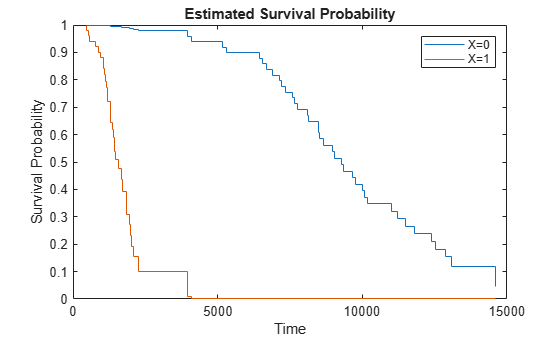# plotSurvival

Plot survival function of Cox proportional hazards model

## Syntax

``plotSurvival(coxMdl)``
``plotSurvival(coxMdl,X)``
``plotSurvival(coxMdl,X,Stratification)``
``plotSurvival(coxMdl,ax,___)``
``plotSurvival(___,'Time',T)``
``graphics = plotSurvival(___)``

## Description

example

````plotSurvival(coxMdl)` plots the baseline survival function of a Cox proportional hazards model `coxMdl`. The survival function at time `t` is the estimated probability of survival until time `t`. The term baseline refers to the survival function at the determined baseline of the predictors. This value is stored in `coxMdl.Baseline`, and the default value is the mean of the data set used for training.```

example

````plotSurvival(coxMdl,X)` plots the survival function when the predictors have the values in `X`. The plot includes one line for each row of `X`.```

example

````plotSurvival(coxMdl,X,Stratification)` plots the survival function for the given value of the stratification variable `Stratification`. You must have one row in `Stratification` for each row in `X`. NoteWhen you train `coxMdl` using stratification variables and pass predictor variables `X`, `plotSurvival` also requires you to pass stratification variables. ```

example

````plotSurvival(coxMdl,ax,___)` plots in the specified graphics axes `ax` using any of the input argument combinations in the previous syntaxes.```

example

````plotSurvival(___,'Time',T)` plots the survival function at times listed in `T`.```
````graphics = plotSurvival(___)` returns an array of `Stair` graphics objects. See Stair Properties.```

## Examples

collapse all

Perform a Cox proportional hazards regression on the `lightbulb` data set, which contains simulated lifetimes of light bulbs. The first column of the light bulb data contains the lifetime (in hours) of two different types of bulbs. The second column contains a binary variable indicating whether the bulb is fluorescent or incandescent; 0 indicates the bulb is fluorescent, and 1 indicates it is incandescent. The third column contains the censoring information, where 0 indicates the bulb was observed until failure, and 1 indicates the observation was censored.

Fit a Cox proportional hazards model for the lifetime of the light bulbs, accounting for censoring. The predictor variable is the type of bulb.

```load lightbulb coxMdl = fitcox(lightbulb(:,2),lightbulb(:,1), ... 'Censoring',lightbulb(:,3));```

Plot the baseline survival function as a function of time `t`, meaning the probability that a light bulb fails after time `t`. By default, the baseline is calculated for the mean of the predictor, which in this case is `mean(lightbulb(:,2))` = `0.5`.

`plotSurvival(coxMdl)`Plot the survival for fluorescent bulbs (predictor = 0) and incandescent bulbs (predictor = 1).

`plotSurvival(coxMdl,[0;1])`To calculate the survival without plotting, use `survival`.

Load the `coxModel` data. (This simulated data is generated in the example Cox Proportional Hazards Model Object.) The model named `coxMdl` has three stratification levels (1, 2, and 3) and a predictor `X` with three categorical values (1, 1/20, and 1/100).

`load coxModel`

Plot the survival for `X = 1` at the three stratification levels.

```c1 = categorical(1); X = [c1;c1;c1]; stratification = [1;2;3]; plotSurvival(coxMdl,X,stratification) xlim([1,30])```Load the `coxModel` data. (This simulated data is generated in the example Cox Proportional Hazards Model Object.) The model named `coxMdl` has three stratification levels (1, 2, and 3) and a predictor `X` with three categorical values (1, 1/20, and 1/100).

`load coxModel`

To enable programmatic editing of a survival plot, create an axes.

```h = figure; axes1 = axes('Parent',h);```

Plot the survival function for the `X` predictor value `categorical(1)` and stratification level 3. This stratification level represents a constant hazard rate. When log-scaled, the resulting survival plot should, therefore, be close to a straight line. Plot for times 1 through 30.

```oo = categorical(1); plotSurvival(coxMdl,axes1,oo,3,'Time',linspace(1,30,300)); axes1.YScale = 'log';```## Input Arguments

collapse all

Fitted Cox proportional hazards model, specified as a `CoxModel` object. Create `coxMdl` using `fitcox`.

Predictors for the model, specified as an array of predictors of the same type used for training `coxMdl`. Each row of `X` represents one set of predictors.

Data Types: `double` | `table` | `categorical`

Stratification level, specified as a variable or variables of the same type used for training `coxMdl`. Specify the same number of rows in `Stratification` as in `X`.

Data Types: `single` | `double` | `logical` | `char` | `string` | `table` | `cell` | `categorical`

Axes for plotting, specified as a graphics axes object.

Times for survival estimates, specified as a real vector. `plotSurvival` sorts the specified times and converts them to a column vector, if necessary. The resulting values are linearly interpolated from times in the training data.

Example: `0:40`

Data Types: `double`